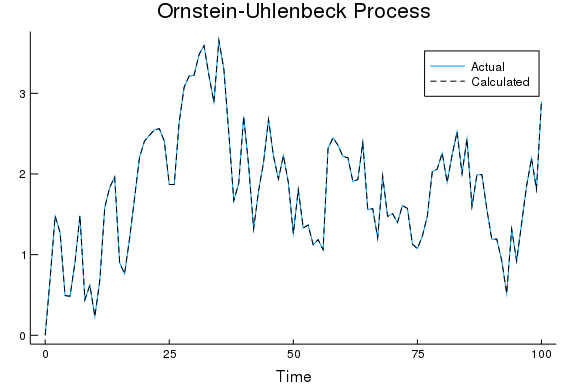Simulating Gaussian processes in julia

# Simulating Gaussian processes in julia

This is my interpretation of the information provided in this tutorial on Noise Problems in the DifferentialEquations package.

# A Simple Wiener Process (White Noise)

A Wiener process is a continuous process that has:

1. Independent increments

2. Gaussian increments

3. Continuous paths, i.e. our Wiener process W is continuous in t

The increments of a Wiener process can be described by the following equation

$\varepsilon_t\cdot\sqrt{dt}$

## Generating a Wiener Process

The first element in the WienerProcess(t0, W0) function is the inital value of time (when we begin the process). The second element is the inital value of our process.

We can also set $dt$ in for our process.

using DifferentialEquations, Plots
W = WienerProcess(0.0,0.0)
dt = 0.1
W.dt = dt
#


## Generate more values

Now we have a Wiener Process object. However, this object doesn't have multiple points and is not plotable. We can add to our process using the code below.

setup_next_step!(W)
for i in 1:100
accept_step!(W,dt)
end


## Plotting the process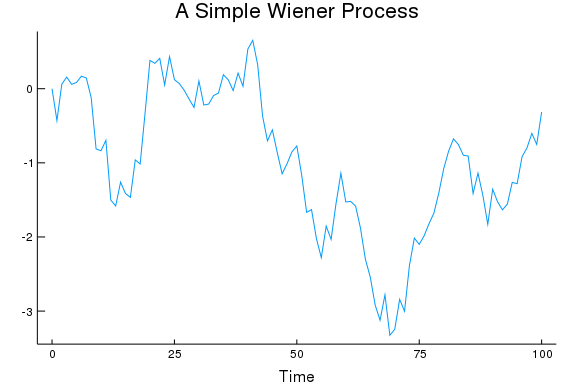# The Brownian Bridge

A Brownian bridge is a Wiener process with a predefined beginning and end point. For the function BrownianBridge(t0,tend,W0,Wend), you input the first time period the last time period and the desired intial and terminal values for the process.

BB = BrownianBridge(0.0, 100, 1.0, 1.0)
BB.dt = 0.1
#


For this Brownian Bridge the initial and terminal value of our process should be 1.0

## Generate more values

Again, we need to add more values to our object

setup_next_step!(BB)
for i in 1:1000
accept_step!(BB,dt)
end


# Plotting the Brownian Bridge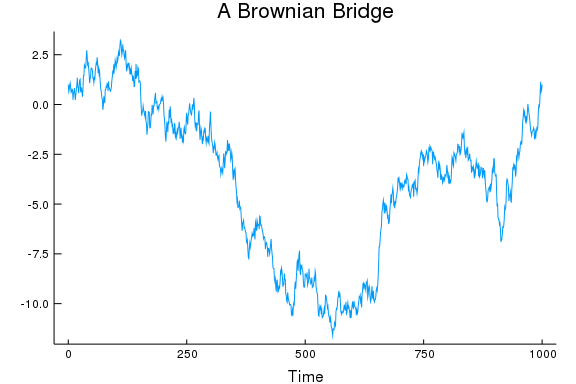# Now we can look at specfic types of Brownian Motion

## Geometric Brownian motion

Geometric Brownian motion can be defined as:

$dx_t = μ x_t dt + σ x_t dW_t$

For the GeometricBrownianMotionProcess() function we need several inputs:

1. $\mu$, our "drift" parameter

2. $\sigma$ our "variance" parameter

3. Intial time period

4. Inital value for our process

μ = 1.0
σ = .8
GB = GeometricBrownianMotionProcess(μ, σ, 0.0, 1.0)
BB.dt = 1.0

1.0


### Generate more values

setup_next_step!(GB)
for i in 1:50
accept_step!(GB,dt)
end


### Plotting Geometric Brownian Motion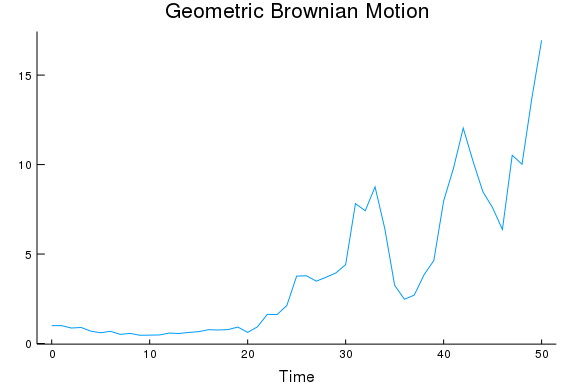## An Ornstein-Uhlenbeck process

The Ornstein-Uhlenbeck process is the continuous time analog of a AR(1) process. We can write this process in the following form,

$dx_t = θ(μ-x_t)dt + σdW_t$

For the OrnsteinUhlenbeckProcess() function we need

1. $\theta$ our "drfit" parameter

2. $\mu$ our "mean" parameter

3. $\sigma$ our vairance parameter

4. Our intial time period t0

5. Our intial value for our process

θ = 1.5
μ = 2.0
σ = 1.5

OU = OrnsteinUhlenbeckProcess(θ, μ, σ, 0.0, 0.0)
OU.dt = 0.1

0.1


### Generate more values

setup_next_step!(OU)
for i in 1:100
accept_step!(OU,dt)
end


### Plotting the Ornstein-Uhlenbeck Process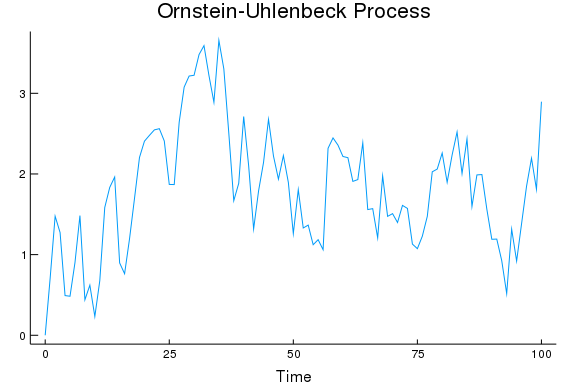### Backing out parameters

Another feature of the DifferentialEquations package is the ability to solve SDE problems and back out the coefficients for our Diffuction process.

OU_prob = NoiseProblem(OU, (0.0,100.0))
solve_OU = solve(OU_prob, dt = 0.12, save_noise=true)
#


Plotting this, and our graph from before we can see that this will give us back thr exact same process we had earlier